﻿ New Modification of Newton Method with Third-Order Convergence for Solving Nonlinear Equations of Type <i>f</i>(0)=0American Journal of Computational and Applied Mathematics

p-ISSN: 2165-8935    e-ISSN: 2165-8943

2016;  6(1): 14-18

doi:10.5923/j.ajcam.20160601.03### New Modification of Newton Method with Third-Order Convergence for Solving Nonlinear Equations of Type f(0)=0

R. Thukral

Padé Research Centre, West Yorkshire, England

Correspondence to: R. Thukral, Padé Research Centre, West Yorkshire, England.
 Email: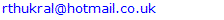Abstract

The objective of this paper is to define a new Newton-type method for finding simple roots of nonlinear equations. It is proved that the new method has the convergence order of three requiring only two function evaluations per iteration. Kung and Traub conjectured that the multipoint iteration methods, without memory based on n evaluations, could achieve maximum convergence order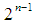, but the new method produces convergence order of three, which is better than expected maximum convergence order of two. Therefore, we show that the conjecture fails for a particular set of nonlinear equations. In fact, we have found that the new method is only usable for zero roots. However, it is observed that our proposed method is very competitive with the third-order methods, thus the new method is very effective in high precision computations.

Keywords: Newton method, Nonlinear equations, Kung-Traub’s conjecture, Optimal order of convergence, Efficiency index, One-point method

Cite this paper: R. Thukral, New Modification of Newton Method with Third-Order Convergence for Solving Nonlinear Equations of Type f(0)=0, American Journal of Computational and Applied Mathematics , Vol. 6 No. 1, 2016, pp. 14-18. doi: 10.5923/j.ajcam.20160601.03.

### 1. Introduction

Finding the root of nonlinear equations is one of important problem in science and engineering [4, 6, 9]. In this paper, we present a new one-point third-order iterative method to find a simple root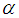of the nonlinear equation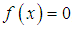, where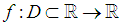for an open interval D is a scalar function. Many higher order variants of the Newton method have been developed based on the Kung and Traub conjecture . Here we present a new iterative method which has a better efficiency index than the second and third order methods given in [2, 4-7, 10]. Hence, the proposed third-order method is significantly better when compared with the established methods.
The classical Newton method for finding simple roots is given by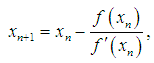(1)
which converges quadratically [4, 6, 9]. For the purpose of this paper, we improve the classical Newton method and construct a new third-order iterative method for finding simple roots of nonlinear equations. The prime motive of this
study is to develop a new class of one-point method for finding simple roots of nonlinear equations. The third-order method presented in this paper only uses two evaluations of the function per iteration. Kung and Traub conjectured that the multipoint iteration methods, without memory based on n evaluations, could achieve optimal convergence order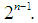In fact, we have obtained a higher order of convergence than the optimal order of convergence suggested by Kung and Traub conjecture . We demonstrate that the Kung and Traub conjecture fails for a particular case, that is when the simple root of a nonlinear equation is equal to zero.
The outline of this paper is as follows: Some basic definitions relevant to the present work are stated in the section 2. In section 3 the new one-point third-order iterative method is constructed and proved. In section 4, four well-established two-point third-order methods are stated, it will demonstrate the effectiveness of the new one-point third-order iterative method. Finally, in section 5, numerical comparisons are made to demonstrate the performance of the new one-point third method.

### 2. Preliminaries

In order to establish the order of convergence of the iterative methods, some of the definitions are stated:
Definition 1 Let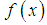be a real function with a simple rootand let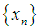be a sequence of real numbers that converge towards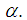The order of convergence p is given by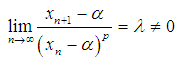(2)
where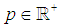and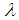is the asymptotic error constant, [1, 4, 6, 9].
Definition 2 Let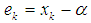be the error in the kth iteration, then the relation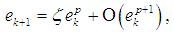(3)
is the error equation. If the error equation exists then p is the order of convergence of the iterative method, [1, 4, 6, 9].
Definition 3 Let r be the number of function evaluations of the iterative method. The efficiency of the iterative method is measured by the concept of efficiency index and defined as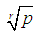(4)
where p is the order of the method, .
Definition 4 (Kung and Traub conjecture) Let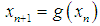define an iterative function without memory with k-evaluations. Then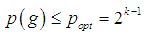(5)
where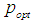is the maximum order .
Definition 5 Suppose that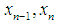and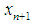are three successive iterations closer to the rootof (1). Then the computational order of convergence may be approximated by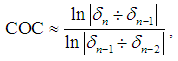(6)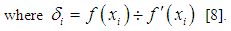### 3. Construction of the One-Point Method and Analysis of Convergence

In this section we define a new one point third-order method for finding simple roots of a nonlinear equation. In fact, the new iterative method is the improvement of the classical Newton method, given by (1). The one-point third-order Newton-type method is expressed by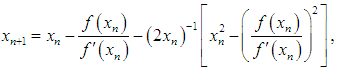(7)
where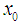is the initial guess and provided that denominators of (7) are not equal to zero. Now, we shall verify the convergence property of the new one-point third-order iterative method (7).
Theorem 1
Let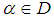be a simple zero of a sufficiently smooth function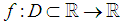for an open interval D. If the initial guess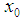is sufficiently close to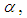then the convergence order of the new one-point iterative method defined by (7) is three.
Proof
Let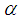be a simple root of, i.e.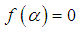and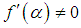, and the error is expressed as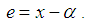Using the Taylor series expansion and taking into account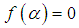, we have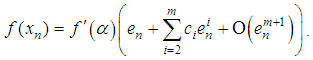(8)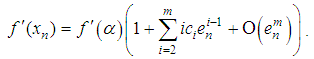(9)
where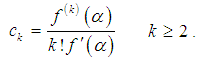(10)
Dividing (8) by (9), we have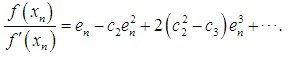(11)
and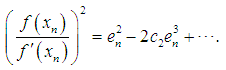(12)
Substituting (11) and (12) in (7), we obtain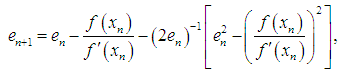(13)
Simplifying (13), yields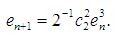(14)
The expression (14) establishes the asymptotic error constant has third-order convergence for the Newton-type method defined by (7).

### 4. The Established Methods

For the purpose of comparison, four two-point third-order methods presented in [2, 5, 7, 10] are considered. Since these methods are well established, we state the essential formulas used to calculate the simple root of nonlinear equations and thus compare the effectiveness of the new one-point third-order method.

#### 4.1. The Weerakoon and Fernando Method

Weerakoon et al.  developed the third-order Newton-type method, given by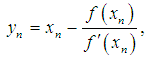(15)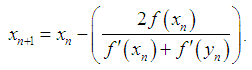(16)

#### 4.2. The Homeier Method

Homeier  presented a third-order Newton-type method, given by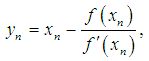(17)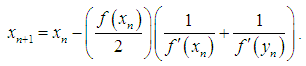(18)

#### 4.3. The Thukral Method

Thukral  developed a third-order variants of the Newton-type method, given by(19)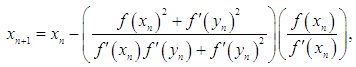(20)

#### 4.4. The Potra-Ptak Method

Potra et al.  developed a third-order variants of the Newton-type method, given by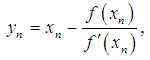(21)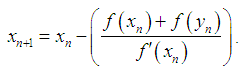(22)

### 5. Numerical Examples

The present one-point third-order method given by (7) is employed to solve nonlinear equations with simple roots. To demonstrate the performance of the new one-point third-order method, ten particular nonlinear equations are used. The consistency and stability of results by examining the convergence of the new iterative methods are determined. The findings are generalised by illustrating the effectiveness of the new method for determining the simple roots of a nonlinear equation. Consequently, estimates are given of the approximate solutions produced by the methods considered and list the errors obtained by each of the methods. The numerical computations listed in the tables were performed on an algebraic system called Maple. In fact, the errors displayed are of absolute value and insignificant approximations by the various methods have been omitted in the following tables.
The new one-point third-order method requires two function evaluations and has the order of convergence three. To determine the efficiency index of the new method, definition 3 shall be used. Hence, the efficiency index of the new iterative method given by (7) is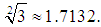and the efficiency index of the two-point third-order methods considered in this paper is given by (16), (18), (20), (22) is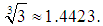It is shown that the efficiency index of the new one-point third-order method is much better than the other similar methods. The test functions and the initial guess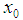are displayed in table 1. The difference between the simple root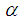and the approximation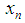for test functions with initial guessare displayed in tables. In fact,is calculated by using the same total number of function evaluations for all methods. Furthermore, the computational order of convergence approximations (COC) are displayed in tables. From the tables we observe that the COCs perfectly coincides with the theoretical result. However, this is the case when initial guess is reasonably close to the sought zeros.
 Table 1. Test functions with simple root α = 0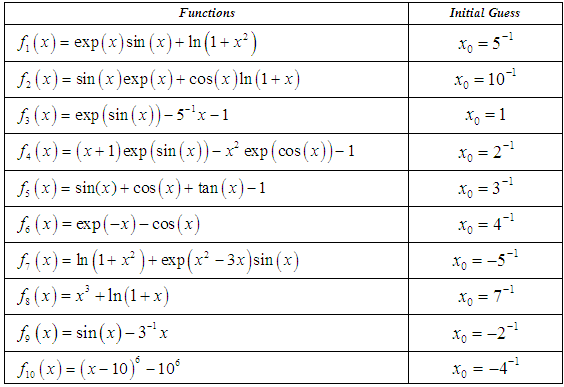Table 2. Performance of various iterative methods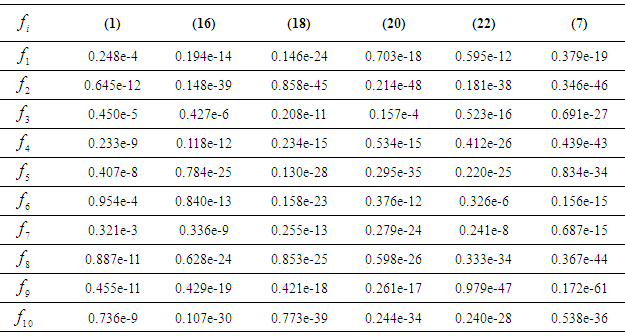Table 3. COC of various iterative methods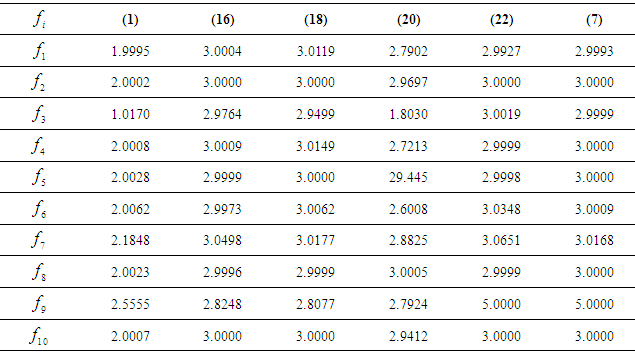### 6. Remarks and Conclusions

In this paper, the performance of the new one-point third-order Newton-type method has been demonstrated. The prime motive of presenting the new iterative method was to improve the classical Newton method. The effectiveness of the new third-order method by showing the accuracy of the simple root of a nonlinear equation have been examined. The main purpose of demonstrating the new Newton-type method for several types of nonlinear equations was purely to illustrate the accuracy of the approximate solution, the stability of the convergence, the consistency of the results and to determine the efficiency of the new iterative method. It has been shown numerically and verified that the new Newton-type method has a convergence order of three. The main advantages are; very high computational efficiency, new method is not limited to the Kung and Traub conjecture, better efficiency index, one point iteration method and very competitive with the two-point third-order methods. Empirically, we have found a drawback of the new one-point third-order method that is only usable when the simple root is zero, hence further investigation is essential.

### References

  W. Gautschi, Numerical Analysis: an Introduction, Birkhauser, 1997.  H. H. H. Homeier, On Newton-type methods with cubic convergence, J. Comput. Appl. Math. 176 (2005) 425-432.  H. Kung, J. F. Traub, Optimal order of one-point and multipoint iteration, J. Assoc. Comput. Math. 21 (1974) 643-651.  M. S. Petkovic, B. Neta, L. D. Petkovic, J. Dzunic, Multipoint methods for solving nonlinear equations, Elsevier 2012.  F. A. Potra, V. Ptak, Nondiscrete introduction and iterative processes, Research notes in Mathematics, vol. 103, Pitman, Boston (1984).  A. M. Ostrowski, Solutions of equations and system of equations, Academic Press, New York, 1960.  R. Thukral, Introduction to a Newton-type method for solving nonlinear equations, Appl. Math. Comput. 195 (2008) 663-668.  R. Thukral, New modifications of Newton-type methods with eighth-order convergence for solving nonlinear equations, J. Adv. Math. Vol 10 (3) (2015) 3362-3373.  J. F. Traub, Iterative Methods for solution of equations, Chelsea publishing company, New York 1977.  S. Weerakoon, T. G. I. Fernando, A variant of Newton’s method with accelerated third-order convergence, Appl. Math. Lett. 13 (2000) 87-93.